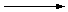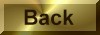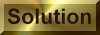Practice Problem 5

An endless number of balanced equations can be written for the reaction between the permanganate ion and hydrogen peroxide in acidic solution to form the manganese (II) ion and oxygen:

 MnO4-(aq) + H2O2(aq)Mn2+(aq) + O2(g)

Use the half-reaction method to determine the correct stoichiometry for this reaction.

Answer

2 MnO4-(aq) + 5 H2O2(aq) + 6 H+(aq)2 Mn2+(aq) + 5 O2(g) + 8 H2O(l)